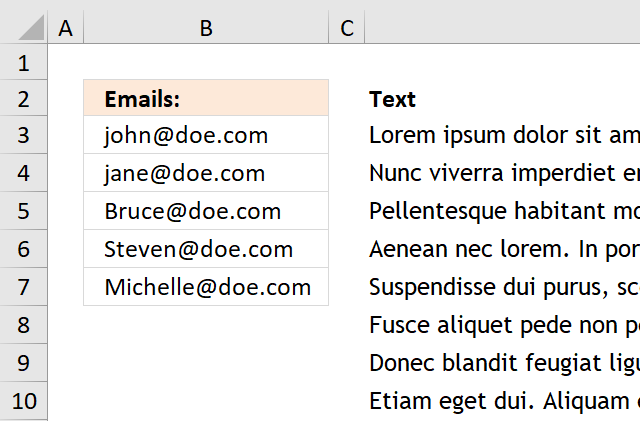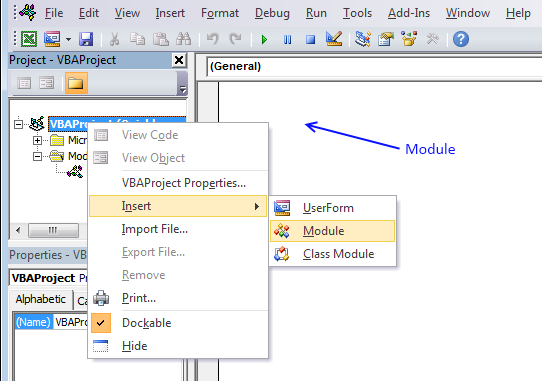Author: Oscar Cronquist Article last updated on January 14, 2019The image above demonstrates a User Defined Function that extracts all words containing a given string that you can specify. In this case it is a @ sign. A User Defined Function is a custom function that you can build yourself in the visual basic editor.

Example, cell range B1:M50 contains random sentences, I have inserted some random emails in this range, see image above.

Array formula in cell range B3:B7:

=FilterWords(D9:O58, "@")

To enter an array formula, type the formula in a cell then press and hold CTRL + SHIFT simultaneously, now press Enter once. Release all keys.

The formula bar now shows the formula with a beginning and ending curly bracket telling you that you entered the formula successfully. Don't enter the curly brackets yourself.

### VBA code

```'Name function
Function FilterWords(rng As Range, str As String) As Variant()
'Declare variables
Dim x As Variant, Wrds() As Variant, Cells_row As Long
Dim Cells_col As Long, Words As Long, y() As Variant
'Redimension variable
ReDim y(0)
'Save values in range to array variable
Wrds = rng.Value
'Iterate through array variable
For Cells_row = LBound(Wrds, 1) To UBound(Wrds, 1)
For Cells_col = LBound(Wrds, 2) To UBound(Wrds, 2)
'Extract words in cell to an array
x = Split(Wrds(Cells_row, Cells_col))
'Iterate through word array
For Words = LBound(x) To UBound(x)
'Check if value in array is equal to the given string
If InStr(x(Words), str) Then
'Save value to another array
y(UBound(y)) = x(Words)
'Increase containers in array by 1
ReDim Preserve y(UBound(y) + 1)
End If
Next Words
Next Cells_col
Next Cells_row
'Decrease containers in array by 1
ReDim Preserve y(UBound(y) - 1)
'Return array
FilterWords = Application.Transpose(y)
End Function
```

### Where to do I copy the code?1. Press Alt-F11 to open visual basic editor
2. Press with left mouse button on Module on the Insert menu
3. Copy and paste the user defined function to module
4. Exit visual basic editor
Note, save your workbook with file extension *.xlsm to keep the user defined function in your workbook.

### Get the Excel fileFilter-emails-from-a-cell-range.xls